# Retro JEE Advanced (Liquid Solutions)

## 8 Questions MCQ Test Chemistry for JEE Advanced | Retro JEE Advanced (Liquid Solutions)

Description
Attempt Retro JEE Advanced (Liquid Solutions) | 8 questions in 10 minutes | Mock test for JEE preparation | Free important questions MCQ to study Chemistry for JEE Advanced for JEE Exam | Download free PDF with solutions
QUESTION: 1

### For a dilute solution containing 2.5 g of a non-volatile non-electrolyte solute in 100 g of water, the elevation in boiling point at 1 atm pressure is 2°C. Assuming concentration of solute is much lower than the concentration of solvent, the vapour pressure (mm of Hg) of the solution is (take Kb = 0,76 K kg mol-1). (2012)

Solution: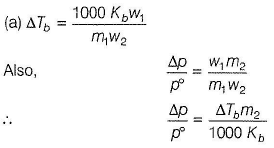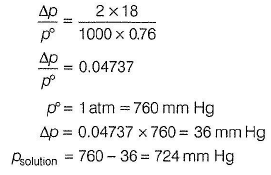QUESTION: 2

### The freezing point (in° C) of solution containing 0.1 g of K3[Fe(CN)6] (mol. wt. 329) in 100 g of water (Kf = 1.86 K kg mol-1) is

Solution: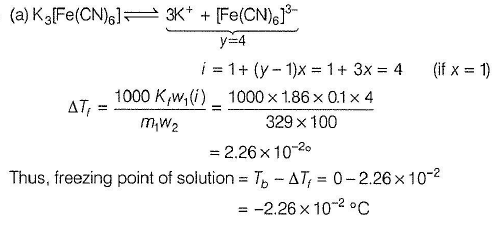QUESTION: 3

### The Henry’s law constant for the solubility of N2 gas in water at 298 K is 1.O x 105 atm. The mole fraction of N2 in air is 0.8. The number of moles of N2 from air dissolved in 10 moles of water of 298 K and 5 atm pressure is (2009)

Solution: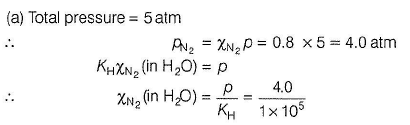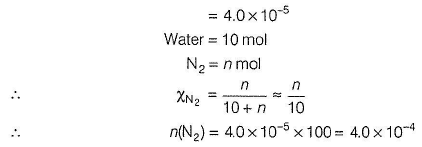QUESTION: 4

Passage

Properties such as boiling point, freezing point and vapour pressure of a pure solvent change when solute molecules are added to get homogeneous solution. These are called colligative properties.
Applications of colligative properties are very useful in day-to-day life. One of its examples is the use of ethylene glycol and water mixture as anti-freezing liquid in the radiator of automobiles.
A solution M is prepared by mixing ethanol and water. The mole fraction of ethanol in the mixture is 0.9.
Given, freezing point depression constant of water

(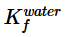) = l . 8 6 K kg mol-1

Freezing point depression constant of ethanol

(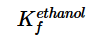) = 2.0 K kg mol-1

Boiling point elevation constant of water

{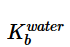) = 0.52 K kg mol-1

Boiling point elevation constant of ethanol

()=1.2 K kg mol-1

Standard freezing point of water =273 K

Standard freezing point of ethanol = 155.7 K

Standard boiling point of water = 373 K

Standard boiling point of ethanol = 351.5 K

Vapour pressure of pure water = 32.8 mm Hg

Vapour pressure of pure ethanol = 40 mm Hg

Molecular weight of water = 18 g mol-1

Molecular weight of ethanol = 46 g mol-1

In answering the following questions, consider the solutions to be ideal dilute solutions and solutes to be non-volatile and non-dissociative.

Q.

The freezing point of the solution M is

Solution:

(d)

Water is solute and ethanol is solvent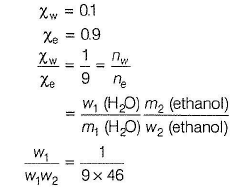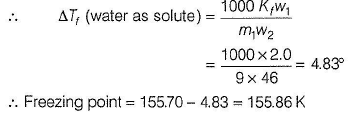QUESTION: 5

Passage

Properties such as boiling point, freezing point and vapour pressure of a pure solvent change when solute molecules are added to get homogeneous solution. These are called colligative properties.
Applications of colligative properties are very useful in day-to-day life. One of its examples is the use of ethylene glycol and water mixture as anti-freezing liquid in the radiator of automobiles.
A solution M is prepared by mixing ethanol and water. The mole fraction of ethanol in the mixture is 0.9.
Given, freezing point depression constant of water

() = l . 8 6 K kg mol-1

Freezing point depression constant of ethanol

() = 2.0 K kg mol-1

Boiling point elevation constant of water

{) = 0.52 K kg mol-1

Boiling point elevation constant of ethanol

()=1.2 K kg mol-1

Standard freezing point of water =273 K

Standard freezing point of ethanol = 155.7 K

Standard boiling point of water = 373 K

Standard boiling point of ethanol = 351.5 K

Vapour pressure of pure water = 32.8 mm Hg

Vapour pressure of pure ethanol = 40 mm Hg

Molecular weight of water = 18 g mol-1

Molecular weight of ethanol = 46 g mol-1

In answering the following questions, consider the solutions to be ideal dilute solutions and solutes to be non-volatile and non-dissociative.

Q.

The vapour pressure of the solution M is

Solution: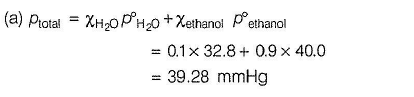QUESTION: 6

Passage

Properties such as boiling point, freezing point and vapour pressure of a pure solvent change when solute molecules are added to get homogeneous solution. These are called colligative properties.
Applications of colligative properties are very useful in day-to-day life. One of its examples is the use of ethylene glycol and water mixture as anti-freezing liquid in the radiator of automobiles.
A solution M is prepared by mixing ethanol and water. The mole fraction of ethanol in the mixture is 0.9.
Given, freezing point depression constant of water

() = l . 8 6 K kg mol-1

Freezing point depression constant of ethanol

() = 2.0 K kg mol-1

Boiling point elevation constant of water

{) = 0.52 K kg mol-1

Boiling point elevation constant of ethanol

()=1.2 K kg mol-1

Standard freezing point of water =273 K

Standard freezing point of ethanol = 155.7 K

Standard boiling point of water = 373 K

Standard boiling point of ethanol = 351.5 K

Vapour pressure of pure water = 32.8 mm Hg

Vapour pressure of pure ethanol = 40 mm Hg

Molecular weight of water = 18 g mol-1

Molecular weight of ethanol = 46 g mol-1

In answering the following questions, consider the solutions to be ideal dilute solutions and solutes to be non-volatile and non-dissociative.

Q.

Water is added to the solution M such that the mole fraction of water in the solution becomes 0.9. The boiling point of this solution is

Solution: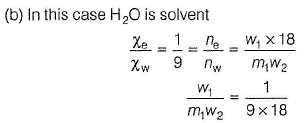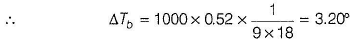∴ Boiling point of solution as water solvent = 373.0 +3.2= 379.2 K

QUESTION: 7

When 20 g of naphthoic acid (C11H8O2 is dissolved in 50 g of benzene {Kf = 1.72 K kg mol-1), a freezing point depression of 2 K is observed. The van’t Hoff factor (i) is

(2007)

Solution: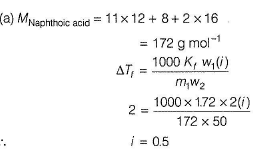QUESTION: 8

The elevation in boiling point, when 13.44 g of freshly prepared CuCI2 are added to one kilogram of water, is (Some useful data, Kb = 0.52 kg K mol-1 molecular weight of CuCI2 =134.4 g).

Solution: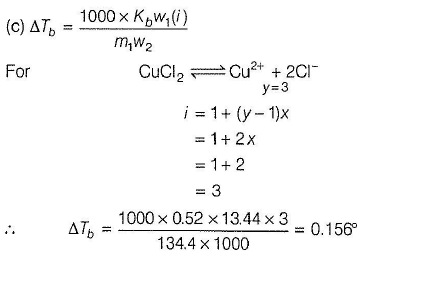Use Code STAYHOME200 and get INR 200 additional OFF Use Coupon Code